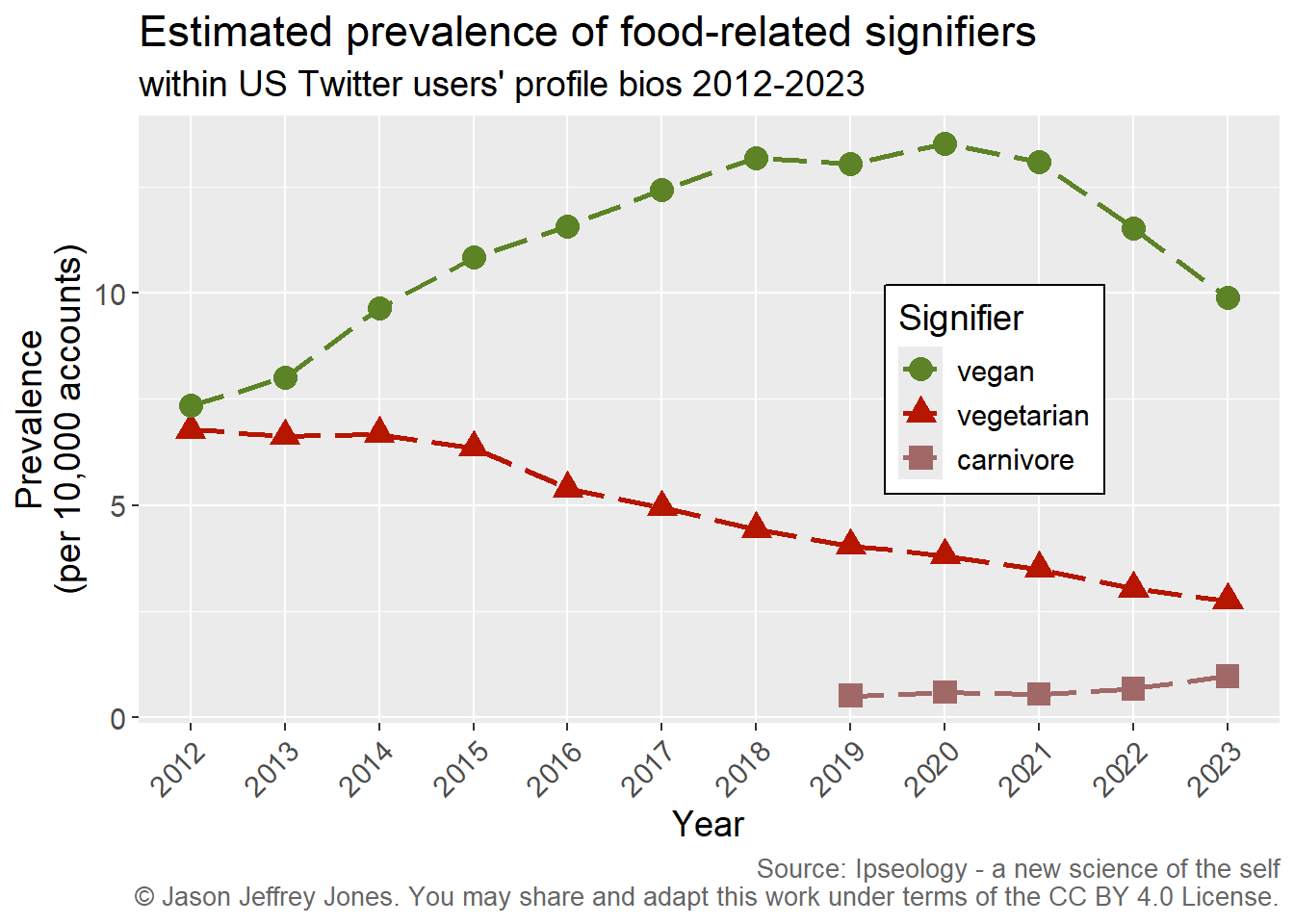# 3Understanding ipseological analysis

## 3.1 Counting is a good place to begin.

How many users include the word `vegan` in their Twitter profile? I can tell you the exact number I have observed for one place and one time. The number is 7,316 for American users in 2012. Call that number the incidence - it’s a raw count.

Now say I want to compare 2012 to 2020. In 2020, the incidence of US `vegan` users was 13,756. Wow, that’s a lot more! But you suspect maybe there were a different number of Twitter users to be observed in 2020 than in 2012, and you are correct. The total number of unique US accounts I observed in 2012 was about 10 million and in 2020 there were about 10.2 million, i.e. 200,000+ more opportunities to observe `vegan`s.

I have included code blocks for those who wish to see implementation details.
Code
``````library(tidyverse)

# Download a csv file containing US data for tokens at annual resolution.

# Become familiar with the data file.
str(tac) # Structure of the data.
tac %>% slice_sample(n = 5)  # Example rows.

# How many users include the word vegan in their Twitter profile?
tac %>% filter(token == "vegan" & obsYear == 2012) # in 2012
tac %>% filter(token == "vegan" & obsYear == 2020) # in 2020
# Note that the numerator column contains the incidence.``````

## 3.2 Prevalence affords comparison.

We need to do some simple math to compare apples-to-apples. We might divide the incidence by the total number of observed accounts to get a proportion. When we do so, we find that 0.000735 proportion of 2012 users include `vegan` versus 0.001351 in 2020.

Code
``````# Calculate ugly proportions.
tac %>% filter(token == "vegan" & (obsYear == 2012 | obsYear == 2020)  ) %>%
mutate(proportion = numerator / denominator)

# Calculate pretty prevalances.
tac %>% filter(token == "vegan" & (obsYear == 2012 | obsYear == 2020)  ) %>%
mutate(prettyPrevalence = round(10000* numerator / denominator) )``````

Yuck. People - even smart ones like you - take more time to compare fractional numbers like these and still make more mistakes. To avoid this unnecessary cognitive hurdle, I consistently express the popularity of signifiers in bios in terms of prevalence per 10,000. Consider Figure 3.1. It shows prevalence for `vegan` among US users for the years 2012-2023. The y-axis has whole number, human-friendly units.

Code
``````# Visualize vegan prevalence over time.
tac %>% filter(token == "vegan") %>%
mutate(finePrevalence = 10000 * numerator / denominator ) %>%
mutate(Signifier = factor(token, levels = c("vegan")) ) %>%
ggplot(aes(x = obsYear, y = finePrevalence, color = Signifier, shape = Signifier)) +
geom_path(linetype = "longdash", linewidth = 1) +
geom_point(size=4) +
scale_x_continuous(limits=c(2012,2023), breaks = 2012:2023, minor_breaks = NULL) +
scale_color_manual(values = c("#5c8326")) +
ggtitle("Estimated prevalence of vegan signifier", "within US Twitter users' profile bios 2012-2023") +
xlab("Year") + ylab("Prevalence\n(per 10,000 accounts)") +
theme(text = element_text(size=16)) +
theme(legend.position = c(0.75, 0.5),
legend.background = element_rect(fill = "white", color = "black")) +
labs(caption = "Source: Ipseology - a new science of the self\n \uA9 Jason Jeffrey Jones. You may share and adapt this work under terms of the CC BY 4.0 License.") +
theme(plot.caption = element_text(size=10, color = "#666666"))``````

Now we have a consistent, simple unit of measurement. Prevalence allows comparison across time and between signifiers. Consider Figure 3.2. Among the observed users, clearly `vegan` increased in popularity while `vegetarian` decreased. `Carnivore` rose above threshold in 2019 and grew through 2023.

(`omnivore` and `pescatarian` never exceeded threshold in any of these years. The threshold to be included in the data was 1 or more per 10,000 after rounding. This eliminates all uniquely-identifying tokens and the long tail of siginifiers used by only tiny fractions of the user population.)

Code
``````# Multiple signifier series.
tac %>% filter(token %in% c("vegan", "vegetarian", "carnivore") ) %>%
mutate(finePrevalence = 10000 * numerator / denominator ) %>%
mutate(Signifier = factor(token, levels = c("vegan", "vegetarian", "carnivore")) ) %>%
ggplot(aes(x = obsYear, y = finePrevalence, color = Signifier, shape = Signifier)) +
geom_path(linetype = "longdash", linewidth = 1) +
geom_point(size=4) +
scale_x_continuous(limits=c(2012,2023), breaks = 2012:2023, minor_breaks = NULL) +
scale_color_manual(values = c("#5c8326", "#B61500", "#a16868") ) +
ggtitle("Estimated prevalence of food-related signifiers", "within US Twitter users' profile bios 2012-2023") +
xlab("Year") + ylab("Prevalence\n(per 10,000 accounts)") +
theme(text = element_text(size=16)) +
theme(legend.position = c(0.75, 0.55),
legend.background = element_rect(fill = "white", color = "black")) +
labs(caption = "Source: Ipseology - a new science of the self\n \uA9 Jason Jeffrey Jones. You may share and adapt this work under terms of the CC BY 4.0 License.") +
theme(plot.caption = element_text(size=10, color = "#666666"))``````Figure 3.2: Prevalence of vegan, vegetarian and carnivore over time. Note that we have measured the popularity of signifiers consistently, persistently and precisely.